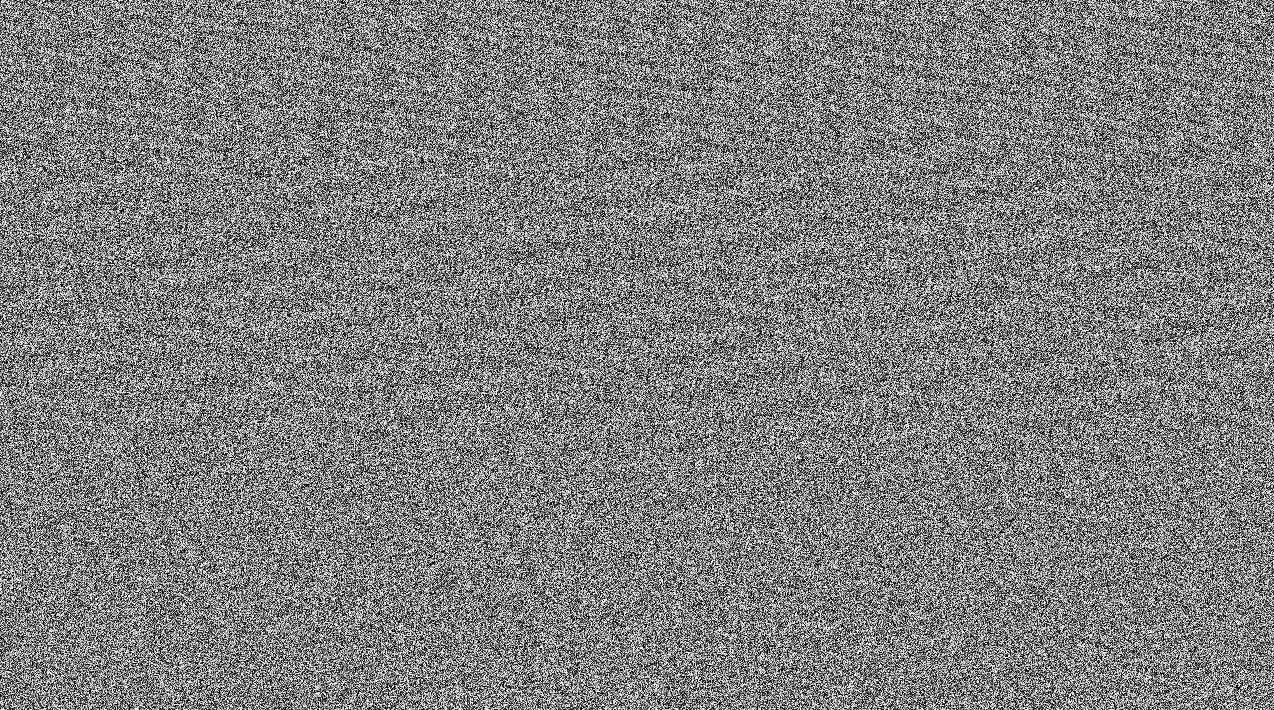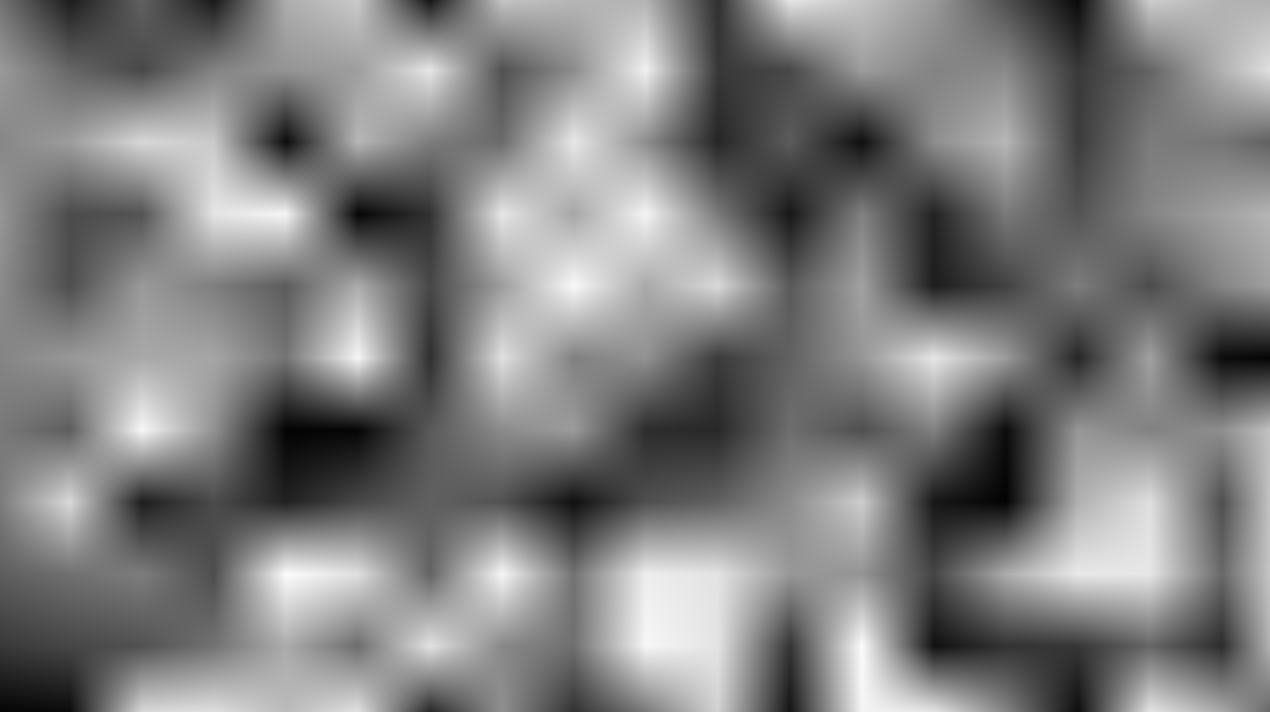A semi-curated blog of computer graphics and rendering.

Hash function is instrumental in making other advanced noises, such as Perlin noise, Worley noise, etc. As it turns out, although undesirable in other parts of signal processing world, noise is very important in computer graphics.

## Hash function

To make some noise, we have to understand the hash function. I recommend The Book of Shaders, which explains this quite well. (Actually it explains Perlin noise as well.) There is also another post on white noise (and other forms of noise), written by XorDev, and you can check it out here. But since this is my post, I will also explain it anyways.

Hash functions are things that, given an input value, spits out one or more random values, usually ranging from 0 to 1. In mathematical terms, that means $$f(x) \rightarrow \mathbb{R}^n, x \in \mathbb{R}^m$$. The result usually doesn’t make any sense, since it’s all random. Here’s how it looks like when $$n = 2, m = 2$$, aka the result of a 2D hash function:Looks a little bit like the telly when there’s nothing on, am I right? Anyway, here’s how to cook one up:

1. Take an input number. If this is a high dimensional hash function, take an input vector.
2. Multiply the input number with some other random number. If the input is a vector, get the dot product instead.
3. Pass the result into a sine wave, and multiply the product with a huge number.
4. Take the fractional part as the final hash result.

Here’s how it looks like in GLSL:

float hash(vec2 uv)
{
return fract(sin(7.289 * uv.x + 11.23 * uv.y) * 23758.5453);
}


## Value Noise

Now, this is all fine and good, but what use does it have? Well, we can create a noise named value noise, using the hash function we implemented above.

Value noise really is just a lower-frequency, interpolated version of the hash function. Here’s how we can make one:

1. Get the input vector/scalar $$v \in \mathbb{R}^n$$ and take the floor result (we can multiply the input first if we want higher/lower frequency result)
2. Sample the $$n^2$$ corners of the hash function
3. Linearly interpolate them.

Here’s how that looks like in GLSL code:

float valueNoise(vec2 uv) {
vec2 u = floor(uv);
vec2 f = fract(uv);
float a = hash(u);
float b = hash(u + vec2(1.0, 0.0));
float c = hash(u + vec2(0.0, 1.0));
float d = hash(u + vec2(1.0, 1.0));
return mix(mix(a, b, f.x),
mix(c, d, f.x), f.y);
}


Zooming out, we can see that indeed, value noise is just a low-frequency version of good ol’ hash function:## But Why Do We Need Those?

I have always been amazed by Inigo Quilez’s Clouds shader. Take a look at this:All of these, the clouds, the sun, in 286 lines of shader code. How did he make it? The clouds need to be mathematically defined or stored in the GPU somehow. As it turns out, the cloud is just a simple 3D Perlin noise texture, then passed through a noise function called fractal Brownian motion proposed by Inigo Quilez himself to produce a volumetric cloud-like look. I have written a shader using iq’s as reference, but I replaced the 3D texture by using a 3D Perlin noise function and calculated the noise value on the fly. You can check it out here.

It was until then do I realized, that the world of mathematics have beauty hidden inside beyond measure. I love math, even though I absolutely suck at it.